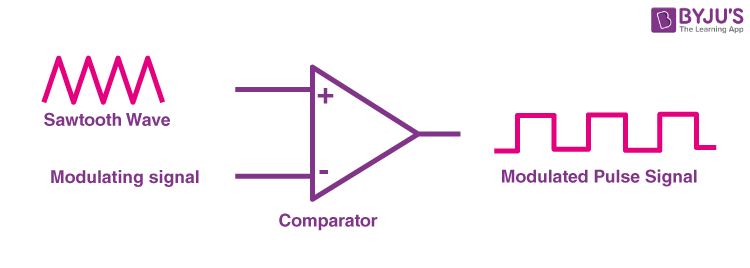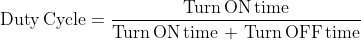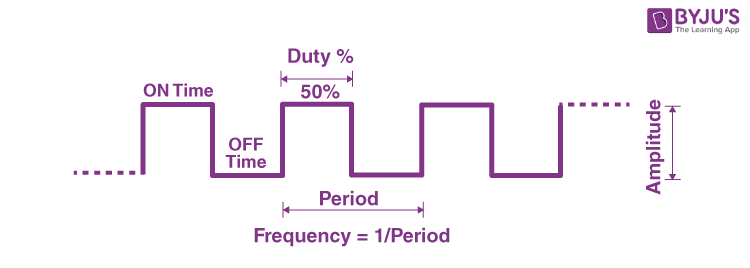# Pulse Width Modulation

In power electronics, pulse width modulation is a proven effective technique that is used to control semiconductor devices. Pulse width modulation or PWM is a commonly used control technique that generates analog signals from digital devices such as microcontrollers. The signal thus produced will have a train of pulses, and these pulses will be in the form of square waves. Thus, at any given time, the wave will either be high or low. Let us learn more about pulse width modulation in this article.

## What is Pulse Width Modulation?

Pulse width modulation reduces the average power delivered by an electrical signal by converting the signal into discrete parts. In the PWM technique, the signal’s energy is distributed through a series of pulses rather than a continuously varying (analog) signal.

### How is a Pulse Width Modulation Signal generated?

A pulse width modulating signal is generated using a comparator. The modulating signal forms one part of the input to the comparator, while the non-sinusoidal wave or sawtooth wave forms the other part of the input. The comparator compares two signals and generates a PWM signal as its output waveform.If the sawtooth signal is more than the modulating signal, then the output signal is in a “High” state. The value of the magnitude determines the comparator output which defines the width of the pulse generated at the output.

## Important Parameters associated with PMW signal

### Duty Cycle of PMW

As we know, a PWM signal stays “ON” for a given time and stays “OFF” for a certain time. The percentage of time for which the signal remains “ON” is known as the duty cycle. If the signal is always “ON,” then the signal must have a 100 % duty cycle. The formula to calculate the duty cycle is given as follows:The average value of the voltage depends on the duty cycle. As a result, the average value can be varied by controlling the width of the “ON” of a pulse.

### Frequency of PMWThe frequency of PMW determines how fast a PMW completes a period. The frequency of a pulse is shown in the figure above.

The frequency of PMW can be calculated as follows:### Output Voltage of PWM signal

The output voltage of the PWM signal will be the percentage of the duty cycle. For example, for a 100% duty cycle, if the operating voltage is 5 V then the output voltage will also be 5 V. If the duty cycle is 50%, then the output voltage will be 2.5 V.

There are three conventional types of pulse width modulation technique and they are named as follows:

• Trail Edge Modulation – In this technique, the signal’s lead edge is modulated, and the trailing edge is kept fixed.
• Lead Edge Modulation – In this technique, the signal’s lead edge is fixed, and the trailing edge is modulated.
• Pulse Center Two Edge Modulation – In this technique, the pulse centre is fixed and both edges of the pulse are modulated.

## Applications of Pulse Width Modulation

Due to the high efficiency, low power loss, and the PWM technique’s ability to precisely control the power, the technique is used in a variety of power applications. Some of the applications of PWM are as follows:

• The pulse width modulation technique is used in telecommunication for encoding purposes.
• The PWM helps in voltage regulation and therefore is used to control the speed of motors.
• The PWM technique controls the fan inside a CPU of the computer, thereby successfully dissipating the heat.
• PWM is used in Audio/Video Amplifiers.

• PWM technique prevents overheating of LED while maintaining its brightness.
• PWM technique is accurate and has a fast response time.
• PWM technique provides a high input power factor.
• PWM technique helps motors generate maximum torque even when they run at lower speeds.

The above were some of the advantages of the Pulse width modulation technique, now let us look at some of the disadvantages:

• As the PWM frequency is high, switching losses are considerably high.
• It induces Radio Frequency Interference (RFI).

## Frequently Asked Questions on Pulse Width Modulation

### What is Pulse Width Modulation technique?

Pulse width modulation or PWM is a commonly used control technique that generates analog signals from digital devices such as microcontrollers. In PWM technique, the signal’s energy is distributed through a series of pulses rather than a continuously varying (analog) signal.

### Why is Pulse Width Modulation used?

Pulse width modulation is an effective method that is used to control the amount of power delivered to a load without dissipating any wasted power.

### What is the difference between the duty cycle and the frequency of a PWM signal?

Frequency of a PWM signal describes how often the signal switches between high and low voltages. Frequency is expressed in the unit of Hertz. Duty cycle is the percentage of time the signal stays at a high voltage. It is expressed as the percentage of the period.

### What are the different types of Pulse Width Modulation?

The three conventional types of pulse width modulation are a) Trail Edge Modulation b) Lead Edge Modulation c) Pulse Center Two Edge Modulation.

### What are some applications of Pulse Width Modulation?

Owing to its high efficiency, low power loss and the ability of the PWM technique to precisely control the power, the technique is used in a variety of power applications: a) They are used in telecommunication for encoding purposes b) They are used in Audio/Video amplifiers.

Stay tuned to BYJU’S and FALL IN LOVE WITH LEARNING!